## Calculate the induced electric field (in V/m) in a 52-turn coil with a diameter of 17 cm that is placed in a spatially uniform magnetic fiel

Question

Calculate the induced electric field (in V/m) in a 52-turn coil with a diameter of 17 cm that is placed in a spatially uniform magnetic field of magnitude 0.45 T so that the face of the coil and the magnetic field are perpendicular. This magnetic field is reduced to zero in 0.10 seconds. Assume that the magnetic field is cylindrically symmetric with respect to the central axis of the coil. (Enter the magnitude.)

in progress 0
6 months 2021-08-22T05:49:02+00:00 1 Answers 2 views 0

## Answers ( )

the induced electric field is 9.95 V/m

Explanation:

Given the data in the question;

Number of turns N = 52

Diameter of coil D = 17 cm = 0.17 m

Radius r = D/2 = 0.17/2 = 0.085 m

Now,

cross-section area A of the coil  = πr²

A = π × ( 0.085 m )²

A = 0.0227 m²

Also given that;

Initial magnetic field B₁ = 0.45 T

Final magnetic field B₂ = 0

∴ change in magnetic field ΔB = B₁ – B₂ = 0.45 T – 0 = 0.45 T

Time taken dT = 0.10 seconds

Now, we know that;

Induced emf ∈ = N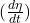where η = BAcosθ

We know that, magnetic field is cylindrically symmetric, coil is also perpendicular to magnetic field.

Hence, the angle between B & A is 0°

∴ θ = 0°

Induced emf ε = N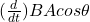we substitute

ε = N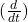(BAcos0°)

A is constant and cos0° = 1

so

ε = NA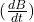We now substitute in our values;

ε = 52 × 0.0227 m² ×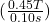ε  =  5.3118 V

we know that, from the relation between electric and emf

ε = ∫∈.dl or ε = ∈∫dl         { for coil; ∫dl = πD }

so we have;

ε = ∈πD

solve for ∈

∈ = ε/πD

we substitute

∈ = 5.3118 V / ( π × 0.17 m )

∈ = 9.95 V/m

Therefore, the induced electric field is 9.95 V/m